# ProFit: Making a Complex Model Image

#### 2019-11-11

Get the latest version of ProFit:

library(devtools)
install_github('ICRAR/ProFit')

First load the libraries we need:

library(ProFit)

## A Simple Model Structure

It is possible to use the fast low level interface to the profitMakeModel function directly. The example below will generate a 100x100 image matrix, and will run in ~5 microseconds:

ExampleImage0=profitMakeModel(list(sersic=list(xcen=50, ycen=50, re=4, nser=4, ang=30, axrat=0.5)))
str(ExampleImage0)
## List of 3
##  $x: num [1:100] 0.5 1.5 2.5 3.5 4.5 5.5 6.5 7.5 8.5 9.5 ... ##$ y: num [1:100] 0.5 1.5 2.5 3.5 4.5 5.5 6.5 7.5 8.5 9.5 ...
##  $z: num [1:100, 1:100] 1.05e-13 1.12e-13 1.18e-13 1.25e-13 1.32e-13 ... The structure is a pure image matrix. It is possible to use base image to view this matrix, but the defaults will make it look pretty dreadful, hard to comprehend, and will be slow to actually plot: image(ExampleImage0)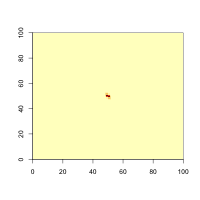We can make a much better image of this model easily using magimage, which chooses useful scaling for astronomical images: magimage(ExampleImage0)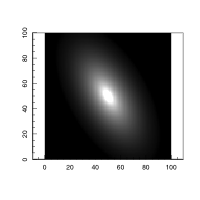We are not limited to simpel single Sersic profiles, highly complex multi-component systems can also be constructed. ## A Multi-Component Structure First we need to setup a legal model structure, with each component type being a separate list. Whilst this seems more fiddly than before, it allows us to make very complex structures and images very easily (i.e. it scales with complexity very well). The top level list can be called whatever you like - model is recommended. The model can contain any number of the following components: sersic / coresersic / moffat / king / brokenexp / pointsource / sky. Here is a legal model structure containing 2 Sersic components, 3 stars (point sources) and a sky pedestal: model1=list( sersic=list( xcen=c(180, 60), ycen=c(90, 10), mag=c(15, 13), re=c(14, 5), nser=c(3, 10), ang=c(46, 80), axrat=c(0.4, 0.6), box=c(0.5,-0.5) ), pointsource=list( xcen=c(34.3,10.1,150), ycen=c(74.2,120.5,130.4), mag=c(10,13,16) ), sky=list( bg=3e-12 ) ) With this structure we can make a simple model using profitMakeModel: ExampleImage1=profitMakeModel(modellist=model1, dim=c(200,200)) str(ExampleImage1) ## List of 3 ##$ x: num [1:200] 0.5 1.5 2.5 3.5 4.5 5.5 6.5 7.5 8.5 9.5 ...
##  $y: num [1:200] 0.5 1.5 2.5 3.5 4.5 5.5 6.5 7.5 8.5 9.5 ... ##$ z: num [1:200, 1:200] 3.57e-11 3.70e-11 3.83e-11 3.97e-11 4.12e-11 ...

Next we can make a greyscale image of our requested model structure:

magimage(ExampleImage1)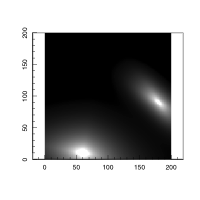Notice there are no stars and the models look very sharp - this is because we have not specified a PSF with which to convolve the image. We can use profitPointSource to create a default circular Gaussian PSF with FWHM=2 pixels:

ExamplePSF=profitMakePointSource()
ExamplePSF=ExamplePSF/sum(ExamplePSF)
ExampleImagePSF1=profitMakeModel(modellist=model1, psf=ExamplePSF, dim=c(200,200))
magimage(ExampleImagePSF1)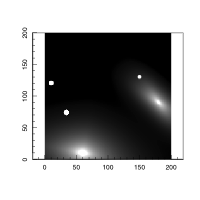That looks much more realistic. The convolution adds a modest ~15 ms to the run time, as the PSF is quite small.

You can also specify the PSF as a Sersic model - specifically a Gaussian, where nser=0.5 and re=FWHM/2~1.17sigma:

model1a=model1
model1a$psf=list(sersic=list(nser=0.5,mag=0,re=1,axrat=1,ang=0)) ExampleImagePSF1A=profitMakeModel(modellist=model1a, psf=ExamplePSF, dim=c(200,200)) Note we are still using the PSF image to convolve the Sersic models, but we use the PSF model to create more accurate images of the point sources. This is because we are integrating the flux in the point source more accurately than by interpolating a static PSF image. We can now easily make a classic bulge+disk system where the two Sersic model components sit on top of each other: modelBD=list( sersic=list( xcen=c(100, 100), ycen=c(100, 100), mag=c(14, 12), re=c(2, 15), nser=c(4, 1), ang=c(0, 60), axrat=c(1, 0.3), box=c(0,0) ) ) ExampleImage=profitMakeModel(modellist=modelBD, psf=ExamplePSF, dim=c(200,200)) magimage(ExampleImage)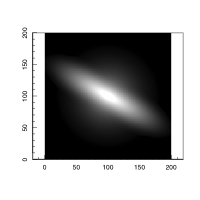The cuspy bulge is evident in the centre of the image, and you can see the dull glow of its large radii wings too (without a truncation high-Nser systems both have a cuspy core and Lorentzian wings that extend off to large radii). Note that the accuracy of convolution is limited by the pixel dimensions relative to the PSF. In this case, the PSF is quite small, so for accurate convolution, we need to sample the model more finely: Finesample=3L FinePSFmodel=model1a$psf
FinePSFmodel$sersic$re=FinePSFmodel$sersic$re*Finesample
ExamplePSFFine=profitMakePointSource(modellist=FinePSFmodel, image=matrix(0,27,27))
ExampleImageFine=profitMakeModel(modellist=modelBD, dim=c(200,200), finesample=Finesample, psf=ExamplePSFFine)

We can see how much of a difference this makes and where:

magimage(ExampleImageFine$z-ExampleImage$z)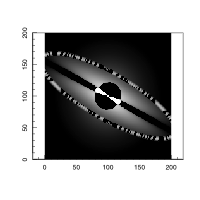maghist((ExampleImageFine$z-ExampleImage$z)/ExampleImageFine$z,xlim=5) ##  "Summary of used sample:" ## Min. 1st Qu. Median Mean 3rd Qu. Max. ## -1.198e-06 4.023e-07 5.753e-07 6.545e-07 8.259e-07 2.354e-06 ##  "sd / 1-sig / 2-sig range:" ##  4.454124e-07 3.280606e-07 1.074342e-06 ##  "Using 27106 out of 40000 (67.77%) data points (3242 < xlo & 9652 > xhi)"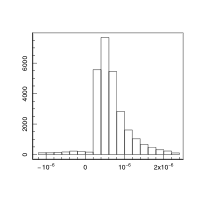This fine sampling step required manual resampling of the PSF by rescaling re and enlarging the PSF image. When fitting models, profitSetupData will handle these steps automatically. ## Make a complex mock image We can use profitMakeModel to make more complex image structure very easily. The example model below will randomly produce 20 Sersic profiles, 10 PSFs and a sky background: model2=list( sersic=list( xcen=runif(20,0,200), ycen=runif(20,0,200), mag=runif(20,15,20), re=runif(20,1,100), nser=runif(20,0.5,8), ang=runif(20,0,180), axrat=runif(20,0.3,1), box=runif(20,-0.3,0.3) ), pointsource=list( xcen=runif(10,0,200), ycen=runif(10,0,200), mag=runif(10,15,20) ), sky=list( bg=3e-12 ) ) As before we can run this through profitMakeModel and then plot the image. This should run in ~0.5 second, which means we are scaling well with our more complex model: system.time(ExampleImagePSF2<-profitMakeModel(modellist=model2, psf=ExamplePSF, dim=c(200,200))) ## user system elapsed ## 0.338 0.001 0.339 magimage(ExampleImagePSF2, hi=1)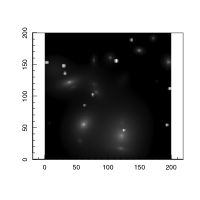We can try a bigger image (more like a typical astronomy image) but this will take longer to generate (~3 seconds). However, this is much faster than the naive 1x(1000/200)^2=25 seconds that we might expect. This is because the lower level C++ routine adaptively adjusts to calculate the pixel integrals. Pixels near the centre need more subdivisions to accurately determine the flux, whereas at large radii typically fewer calculations are required. Hence generating a much bigger image does not directly scale the computation time. model3=list( sersic=list( xcen=runif(20,0,1000), ycen=runif(20,0,1000), mag=runif(20,15,20), re=runif(20,1,100), nser=runif(20,0.5,8), ang=runif(20,0,180), axrat=runif(20,0.3,1), box=runif(20,-0.3,0.3) ), pointsource=list( xcen=runif(10,0,1000), ycen=runif(10,0,1000), mag=runif(10,15,20) ), sky=list( bg=3e-12 ) ) system.time(ExampleImagePSF3<-profitMakeModel(modellist=model3, psf=ExamplePSF, dim=c(1000,1000))) ## user system elapsed ## 1.800 0.003 1.805 magimage(ExampleImagePSF3)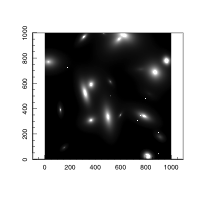A faster image can be generated if the remax flag is turned on. With remax=10 it only takes ~2 seconds to make the model image. This might be accurate enough for many simulation purposes and can be a factor of 2 faster. system.time(profitMakeModel(modellist=model3, psf=ExamplePSF, dim=c(1000,1000), remax=10)) ## user system elapsed ## 1.067 0.008 1.076 It is easy to add together model images using profitAddMats’, giving flexibility in how mock images are made: ExampleImageAdd=profitAddMats(ExampleImagePSF3$z, ExampleImagePSF2\$z, c(300,400))
magimage(ExampleImageAdd)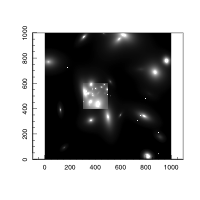In this example it is evident that there is a lot of low surface brightness flux in ExampleImagePSF2’, so a much bigger image should be made to remove the flux discontinuity at the edges of the 200x200 sub-image.